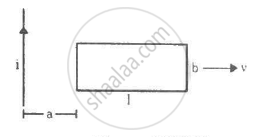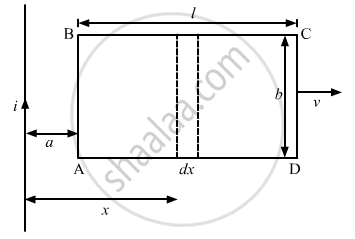Advertisement Remove all ads

# A Rectangular Metallic Loop of Length L And Width B Is Placed Coplanarly with a Long Wire Carrying a Current I (Figure). - Physics

Sum

A rectangular metallic loop of length l and width b is placed coplanarly with a long wire carrying a current i (figure). The loop is moved perpendicular to the wire with a speed vin the plane containing the wire and the loop. Calculate the emf induced in the loop when the rear end of the loop is at a distance a from the wire. solve by using Faraday's law for the flux through the loop and also by replacing different segments with equivalent batteries.Advertisement Remove all ads

#### Solution

Consider an element of the loop of length dx at a distance x from the current-carrying wire.The magnetic field at a distance x from the the current-carrying wire is given by

$B = \frac{\mu_0 i}{2\pi x}$

Area of the loop = bdx

Magnetic flux through the loop element:-

$d\phi = \frac{\mu_0 i}{2\pi x}bdx$

The magnetic flux through the loop is calculated by integrating the above expression.

Thus, we have

$\phi = \int\limits_a^{a + l} \frac{\mu_0 i}{2\pi x}bdx$

$= \frac{\mu_0 i}{2\pi}b \int\limits_a^{a + l} \left( \frac{dx}{x} \right)$

$= \frac{\mu_0 i}{2\pi x}\ln\left( \frac{a + l}{a} \right)$

The emf can be calculated as:-

$e = - \frac{d\phi}{dt} = \frac{d}{dt}\left[ \frac{\mu_0 ib}{2\pi}\log\left( \frac{a + l}{a} \right) \right]$

$= - \frac{\mu_0 ib}{2\pi}\frac{a}{a + l}\left( \frac{va - (a + l) v}{a^2} \right) .............\left( \because \frac{da}{dt} = v \right)$

$= \frac{\mu_0 ib}{2\pi}\frac{a}{a + l}\frac{vl}{a^2}$

$= \frac{\mu_0 ib vl}{2\pi (a + l)a}$

Calculation of the emf using the emf method:-

The emf. induced in AB and CD due to their motion in the magnetic field are opposite to each other.

The magnetic field at AB is given by

$B_{AB} = \frac{\mu_0 i}{2\pi a}$

Now,
Length = b
Velocity = v
The emf induced in AB is given by

$e_{AB} = \frac{\mu_0 ivb}{2\pi a}$

The magnetic field at CD is given by

$B_{CD} = \frac{\mu_0 i}{2\pi (a + l)}$

The emf induced in side CD is given by

$e_{CD} = \frac{\mu_0 ibv}{2\pi (a + l)}$

The net emf induced is given by

$e_{net} = \frac{\mu_0 ibv}{2\pi a} - \frac{\mu_0 ibv}{2\pi (a + l)}$

$= \frac{\mu_0 ibl(a + l) - \mu ibva}{2\pi a(a + l)}$

$= \frac{\mu_0 ibvl}{2\pi a(a + l)}$

Is there an error in this question or solution?
Advertisement Remove all ads

#### APPEARS IN

HC Verma Class 11, Class 12 Concepts of Physics Vol. 2
Chapter 16 Electromagnetic Induction
Q 58 | Page 311
Advertisement Remove all ads

#### Video TutorialsVIEW ALL 

Advertisement Remove all ads
Share
Notifications

View all notifications

Forgot password?